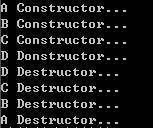# 构造函数和析构函数

## 复制构造函数

1）根据另一个同类型的对象初始化一个对象

string a("123456");
string b(a);


2）在顺序容器的元素初始化和类类型数组元素初始化时，也会默认使用复制构造函数。
3）复制一个对象，将它作为实参传给一个函数或从函数返回时复制一个对象。也就是说，如果函数的形参或返回值是一个类的话，将会调用复制构造函数进行复制。

class Myclass
{
public:
Myclass(int n) { number = n; }
Myclass(const Myclass &other)
{
number = other.number;
cout << "copy";
}
private:
int number;
};

Myclass fun(Myclass p)
{
Myclass temp(p);
return temp;
}

void main()
{

Myclass a(10);
Myclass b(a);
Myclass c = fun(b);
system("pause");
}


## 析构函数

class A {
public:
A() { cout << "A"; }
~A() { cout << "~A"; }
};
class B: public A{
public:
B() { cout << "B"; }
~B() { cout << "~B"; }
};

void f()
{
A *a = new B;
delete a;
getchar();
}
int  main()
{
f();
system("pause");
return 0;
}


# 构造函数与析构函数的调用顺序

## 单继承

class A {
public:
A() { cout << "A"; }
A(const A &a) { cout << "copy"; }
virtual ~A() { cout << "~A"; }

};
class B: public A{
public:
B(A &a):_a(a) { cout << "B"; }
virtual ~B() { cout << "~B"; }
private:
A _a;
};

void f()
{
A(a);
B b(a);
}
int  main()
{
f();
system("pause");
return 0;
}


A A copy B ~B ~A ~A ~A

1）完成对象所占整块内存的开辟，由系统调用构造函数自动完成
2）调用基类的构造函数完成基类成员的初始化
3）派生类中含对象成员，const成员和引用成员的初始化
4）派生类构造函数

## 多继承

class A
{
public:
A() { cout << "A Constructor..." << endl; }
~A() { cout << "A Destructor..." << endl; }
};

class B
{
public:
B() { cout << "B Constructor..." << endl; }
~B() { cout << "B Destructor..." << endl; }
};

class C :public A, public B
{
public:
C() { cout << "C Constructor..." << endl; }
~C() { cout << "C Destructor..." << endl; }
};

class D : public C
{
public:
D() { cout << "D Donstructor..." << endl; }
~D() { cout << "D Destructor..." << endl; }
};

void f()
{
D d;
}

void main()
{
f();
system("pause");
}©️2019 CSDN 皮肤主题: 技术黑板 设计师: CSDN官方博客